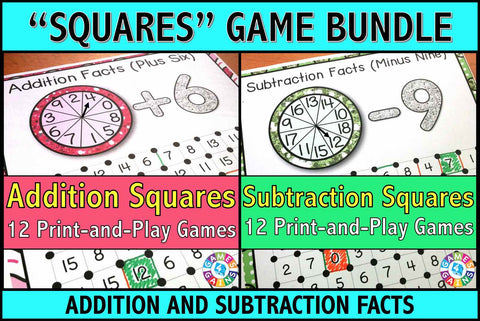## Addition and Subtraction 'Squares' Games Bundle

• \$720
• Save \$0.80

Addition and Subtraction 'Squares' Games Bundle contains our Addition 'Squares' Game and our Subtraction 'Squares' Game for 10% off their individual prices.

When you buy this bundle, you'll receive 24 fun and engaging addition and subtraction games to help students practice their addition and subtraction facts.

Each one-page game gets students practicing a different set of addition or subtraction facts. This means that you can have each student practicing the set of facts that he/she needs the most help with.

These Addition and Subtraction 'Squares' games are so simple to use, and take a minimal amount of prep. Simply print out the game sheet, get a paperclip and fastener, and grab a few markers, and you'll be ready to go!

To play, students will spin the spinner and solve the addition or subtraction problem. Then, they have to find the solution on the game board and draw a line between two of the dots surrounding that number. When a student's line forms a complete square, he/she gets to capture that square. The student with the most squares at the end of the game is the winner!